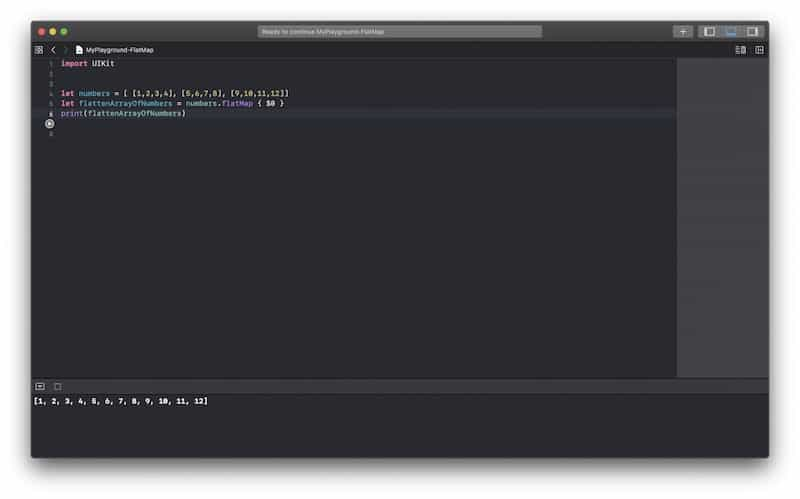# How to Use flatMap in Swift. Code Examples.

In this Swift tutorial, you will learn how to use the flatMap function in Swift which will flatten an Array of Arrays into a single array of elements.

## Given an Array of Arrays

Let’s consider the following Array of Arrays.

`let numbers = [ [1,2,3,4], [5,6,7,8], [9,10,11,12]]`

The above array contains 3 arrays of integers. We can use flatMap function to flatten this array of arrays into a single array of numbers.

## FlatMap Example in Swift

Below is an example of how you can use the flatMap function in Swift.

```let numbers = [ [1,2,3,4], [5,6,7,8], [9,10,11,12]]
let flattenArrayOfNumbers = numbers.flatMap { \$0 }

print(flattenArrayOfNumbers)```

If you run the above code example in Xcode Playground you will get the following result.

`1, 2, 3, 4, 5, 6, 7, 8, 9, 10, 11, 12]`

and here is a screenshot of my Xcode playground with the above Swift code demonstrating how flatMap function works.I hope this code example was helpful to you. If you are interested to learn how the map(_:) function works in Swift, check this blog post: How to use map(_:) in Swift.